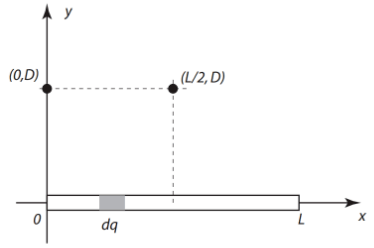# Problem: A total charge Q is distributed uniformly over rod length L. The rod is aligned on the x-axis, with one end at the origin and the other at point x = L.a) calculate electric field at a point (0,D) b) calculate the electric field at point (L/2,D)

###### FREE Expert Solution

This problem required us to calculate the electric field at points (0, D) and at (L/2, D).

Electric field is expressed as:

$\overline{){\mathbf{E}}{\mathbf{=}}\frac{\mathbf{k}\mathbf{q}}{{\mathbf{r}}^{\mathbf{2}}}}$, where is Coulomb's constant, q is the charge, and r is the distance from the point to the charge.

$\overline{){\mathbf{q}}{\mathbf{=}}{\mathbf{\lambda }}{\mathbf{L}}}$, where λ is the line charge density and is the length.

a)

Taking an element of the charge, dq:

$\overline{){\mathbf{d}}{\mathbf{q}}{\mathbf{=}}{\mathbf{\lambda }}{\mathbf{d}}{\mathbf{x}}}$

We now have, dE = dEcosθ(-i) + dE sinθ (j) ........................(1)

Also,

$\begin{array}{rcl}\mathbf{d}\mathbf{E}& \mathbf{=}& \frac{\mathbf{k}\mathbf{d}\mathbf{q}}{{\mathbf{r}}^{\mathbf{2}}}\\ & \mathbf{=}& \frac{\mathbf{k}\mathbf{\lambda }\mathbf{d}\mathbf{x}}{\mathbf{\left(}{\mathbf{D}}^{\mathbf{2}}\mathbf{+}{\mathbf{x}}^{\mathbf{2}}\mathbf{\right)}}\end{array}$

Substituting this to equation (1):

$\begin{array}{rcl}\mathbf{d}\mathbf{E}& \mathbf{=}& \frac{\mathbf{k}\mathbf{\lambda }\mathbf{d}\mathbf{x}}{\mathbf{\left(}{\mathbf{D}}^{\mathbf{2}}\mathbf{+}{\mathbf{x}}^{\mathbf{2}}\mathbf{\right)}}\mathbf{\left[}\mathbf{c}\mathbf{o}\mathbf{s}\mathbf{\theta }\mathbf{\left(}\mathbf{-}\mathbf{i}\mathbf{\right)}\mathbf{+}\mathbf{s}\mathbf{i}\mathbf{n}\mathbf{\theta }\mathbf{\left(}\mathbf{j}\mathbf{\right)}\mathbf{\right]}\end{array}$

91% (66 ratings)###### Problem Details

A total charge Q is distributed uniformly over rod length L. The rod is aligned on the x-axis, with one end at the origin and the other at point x = L.a) calculate electric field at a point (0,D)

b) calculate the electric field at point (L/2,D)